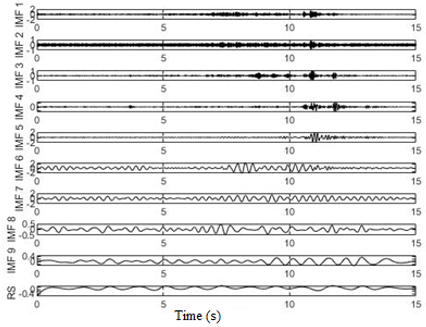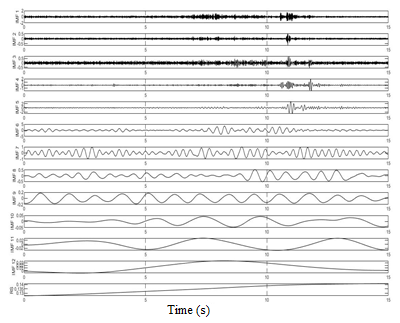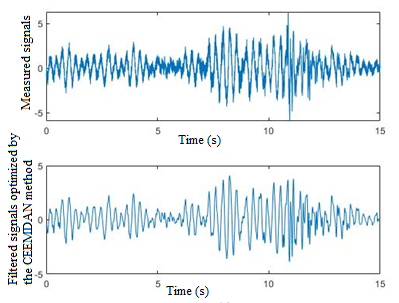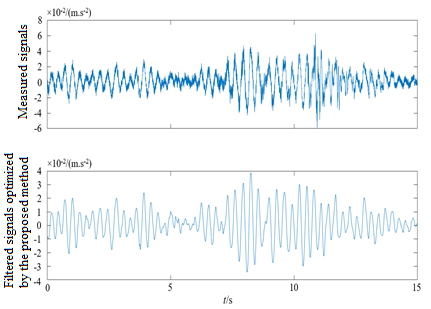# An Adaptive Filtering Method for Bridge Vibration Signals Based on Improved CEEMDAN and Multi-Scale Permutation Entropy

An Adaptive Filtering Method for Bridge Vibration Signals Based on Improved CEEMDAN and Multi-Scale Permutation Entropy
Construction Engineering College, Jilin University, Changchun 130021, China

Changchun Smart City Science and Technology Co. Ltd., Changchun 130000, China

Corresponding Author Email:
gao_xin@jlu.edu.cn
Page:
163-168
|
DOI:
https://doi.org/10.18280/eesrj.080404
17 September 2021
|
Accepted:
10 October 2021
|
Published:
31 December 2021
| Citation

OPEN ACCESS

Abstract:

Aiming at the serious noise of bridge vibration signals in complex environment, this paper proposed an adaptive filtering and denoising optimization method for bridge structural health monitoring. The method took CEEMDAN algorithm as the core, during the step-by-step decomposition of original signals, white noise with opposite signs was added in each stage, meanwhile multi-scale permutation entropy (MPE) was introduced to analyze the mean entropy of each intrinsic mode function (IMF) at different scales, and components with serious noise were eliminated to complete the first filtering; then, in order to optimize the remaining IMFs for signal reconstruction and ensuring the smoothness and similarity of filtering, an optimized reconstruction model was established to complete the second filtering. Compared with the CEEMDAN method, the proposed method could solve the problems of mode mixing and endpoint effect with good completeness, orthogonality, and signal-to-noise ratio. At last, the advantages and application value of the proposed method were verified again by the vibration signal analysis of a real long-span bridge structure.

Keywords:

health monitoring, filtering, bridge, CEEMDAN, decomposition and reconstruction

1. Introduction

When monitoring the health of bridge structures, we need to extract vibration signals of the structures using sensors, however, under the complex excitations of the environment, the extracted vibration signals often contain a lot of random noise. Therefore, how to remove noise to the greatest extent while ensuring good completeness of the vibration signals is the essential issue to be solved.

In view of such problems, Yeh et al.  proposed the Complementary Ensemble Empirical Mode Decomposition (CEEMD) method which reduces the reconstruction errors by adding white noise with opposite signs into the original signals for multiple times. Compared with the applications of Ensemble Empirical Mode Decomposition (EEMD) [2-4], the CEEMD method has a wider application range, such as in fields of instrument failure detection [5-8], financial law prediction [9-13], and environment model amendments [14, 15], etc. However, due to the addition of paired white noise, the amount of calculation will increase and the calculation efficiency will decrease. To cope with this problem, Torres et al.  proposed the Complete Ensemble Empirical Mode Decomposition with Adaptive Noise (CEEMDAN) with adaptive white noise, which not only solves the low calculation efficiency of CEEMD, but also enhances the self-adaptability of signal decomposition. As for the endpoint effect, there are many methods [17-19] to suppress it. CEEMDAN is more effective in aspects such as mechanical failure detection [20-25] and model optimization [26-28]; Patricio Fuentealba et al.  used CEEMDAN to assess the condition of the fetus during delivery; Yao and Liu  applied MPE to the identification of EEG signals; Hu et al.  combined CEEMDAN with MPE to identify the state of ball mills under different loads; Wang et al.  proposed an optimized filtering method that combines CEEMDAN with MPE. However, the completeness of the CEEMDAN algorithm itself needs to be strengthened further.

In this paper, the CEEMDAN algorithm was optimized, the noise was retrieved and eliminated by MPE, and brought into the optimized reconstruction model, then endpoint symmetrical extension was performed to suppress the endpoint effect. Compared with the CEEMDAN algorithm, the proposed method showed better completeness and higher calculation efficiency, and the problems of mode mixing and endpoint effect had been improved effectively. The processing of simulated signals and measured signals indicated that the proposed method can realize the extraction of real information of the signals.

2. Core Principles

2.1 The optimized CEEMDAN algorithm

Based on the CEEMDAN  algorithm, this paper proposed an optimized algorithm, its calculation principles are as follows:

First, the operator Ek( ) represents the endpoint symmetric extension of the k-th modal component generated by EMD (Empirical Mode Decomposition); n represents the number of added noise pairs; ±a represents the amplitude of each noise pairs, then the expression of the added noise is:

$T_{x i}=\mathrm{t}+\mathrm{a} p_{i}$               (1)

$T_{y i}=\mathrm{t}-\mathrm{a} p_{i}$                (2)

In Formulas (1) and (2), t is the original signal, pi is the Gaussian white noise added for the i-th time, Txi and Tyi are respectively the results of adding positive noise and negative noise for the i-th time, where i=1,2,... n. Then, Txi and Tyi were subject to EMD and decomposed into IMFxi and IMFyi, and then were processed by endpoint symmetric extension, and the integrated average of the two was taken as the final decomposed IMF1, namely:

$I M F_{1}=\frac{1}{2 n}\left(\sum_{i=1}^{n} I M F_{x i}+\sum_{i=1}^{n} I M F_{y i}\right)$                   (3)

Suppose IMFk-1 represents the sum of all decomposition results of the previous-level decomposition, taking the current level as an example, there is: IMFk-1= IMF1, and the residual of current level is Rk=t-IMFk-1, then, based on this residual, the next-level decomposition was performed:

$T_{x i}=R_{k}+E_{k}\left(\mathrm{a} p_{i}\right)$                 (4)

$T_{y i}=R_{k}-E_{k}\left(\mathrm{a} p_{i}\right)$                  (5)

$I M F_{k+1}=\frac{1}{2 n}\left(\sum_{i=1}^{n} E_{1}\left(T_{x i}\right)+\sum_{i=1}^{n} E_{1}\left(T_{y i}\right)\right)$                     (6)

Formula (6) was executed repeatedly until the residual satisfies a mean value of 0 or the difference between the number of extreme points and the number of zero points is not more than 2, then the iteration terminates, at this time, if the number of modal components obtained after decomposition is M (residual is not included), then in the above formula, k=1,2,…M-1, and the expression of the final decomposition results is:

$\mathrm{t}=\sum_{i=1}^{\mathrm{M}} I M F_{i}+R_{\mathrm{M}+1}$                  (7)

2.2 MPE

Multi-scale Permutation Entropy (MPE) is an adaptive algorithm proposed by Aziz and Arif , its calculation principles are as follows :

(1) The time series X={x1, x2, x3……xN} with a length of N was subject to coarse graining processing:

$y_{j}^{s}=\frac{1}{s} \sum_{i=(j-1) s+1}^{j s} x_{i}, 1 \leq j \leq \frac{\mathrm{N}}{s}$                  (8)

where, s is the scale factor, and $y_{j}^{s}$ is a multi-scale time series.

(2) $y_{j}^{s}$ was subject to time reconstruction to get:

$Y_{t}^{s}=\left\{y_{t}^{s}, y_{t+\tau}^{s}, \cdots, y_{t+(m-1) \tau}^{s}\right\}$                 (9)

where, m is the embedding dimension, and τ is the delay time. The reconstructed series was re-arranged in ascending order to give a sequence of characteristic symbols:

$S(x)=\left\{j_{1}, j_{2}, \cdots, j_{m}\right\}, x=1,2, \cdots, n \leq m !$                   (10)

where, x is the type of the symbol sequence, and n is the number of sequence types.

(3) Px the probability of each symbol sequence appearing in the m! types of symbol sequences was calculated, then, the MPE of time series X was:

$\operatorname{MPE}(X)=-\sum_{x=1}^{n} P_{x} \ln P_{x}$                     (11)

(4) The MPE was normalized:

$\overline{\operatorname{MPE}(X)}=\operatorname{MPE}(X) / \ln (m !)$                    (12)

In terms of the value assignment of m and τ , in this paper, m took a value of 6, and τ took 1s. Suppose the scale factor s was within [1, 15], the MPE threshold was set to 0.58, so the components with a MPE value greater than 0.58 had been eliminated.

2.3 The optimized reconstruction model of similarity and smoothness

To ensure good similarity and smoothness of the reconstructed signals, the following mathematical model was established .

In terms of the similarity index, suppose the difference between reconstructed signals and original signals was: X={X1,X2,…,XP}, then the similarity S was defined as:

$S=\operatorname{std}(X)=\sqrt{\frac{\sum_{i-1}^{\mathrm{p}}\left(X_{i}-\bar{X}\right)^{2}}{\mathrm{P}-1}}$                  (13)

where, P is the total number of filtering sample points; the smaller the value of S, the better the similarity.

In terms of the smoothness index, suppose Y(a) represents the filtering function, a[a1,an], an=a1+(n-1)t, t is step size, then the left and right curvatures at the point ak(1<k<n) are:

\begin{aligned} K_{a_{k}}^{-} &=\frac{\left|Y^{-}\left(a_{k}\right)^{\prime \prime}\right|}{\left(1+Y^{-}\left(a_{k}\right)^{\prime}\right)^{3 / 2}} \\ K_{a_{k}}^{+} &=\frac{\left|Y^{+}\left(a_{k}\right)^{\prime \prime}\right|}{\left(1+Y^{+}\left(a_{k}\right)^{\prime}\right)^{3 / 2}} \end{aligned}                     (14)

If the curve is smooth and derivable at point ak, then the left and right curvatures of this point are the same, that is:

$\left|Y^{-}\left(a_{k}\right)^{\prime \prime}\right|=\left|Y^{+}\left(a_{k}\right)^{\prime \prime}\right|$                    (15)

Expand the above formula in the difference scheme:

$Y^{-}\left(a_{k}\right)^{\prime \prime}=\frac{Y\left(a_{k}-2 t\right)-2 Y\left(a_{k}-t\right)+Y\left(a_{k}\right)}{t^{2}}$

$Y^{+}\left(a_{k}\right)^{\prime \prime}=\frac{Y\left(a_{k}+2 t\right)-2 Y\left(a_{k}+t\right)+Y\left(a_{k}\right)}{t^{2}}$                      (16)

Combining above formulas, there’s:

$g=Y\left(a_{k}+2 t\right)-Y\left(a_{k}-2 t\right)-2\left[Y\left(a_{k}+t\right)-Y\left(a_{k}-t\right)\right]$                    (17)

In above formula, g is the smoothness at this point, and the smoothness of the entire signal section is G=std(g). The smaller the value of G, the better the smoothness of the reconstructed signal.

The construction of the model needs to balance the two contradictory variables of S and G, so an objective function Z was set, and the minimum filtered signals of Z were taken as the final optimized filtered signals, so the final model was:

$\min Z=0.5 S+0.5 G$                 (18)

3. Analysis of the Vibration Signals of a Real Bridge

3.1 Parameter setting

In order to verify the effectiveness of the proposed method, in the experiment, the vibration singles of a real bridge were respectively subject to the processing of the CEEMDAN method and the proposed method. The parameter setting of the real signals is listed in Table 1.

The specific operating parameters of the two methods are given in Table 2.

Table 1. Parameter information of vibration signals

 Parameter information of real signals Signal frequency 256Hz Cut-off length 15s Number of sample points 3840

Table 2. Vibration signal processing conditions

 The CEEMDAN method The proposed method Amplitude level of the added Gaussian white noise 40% 40% The number of times of adding Gaussian white noise 200(pairs)×2 200(pairs)×2 Number of iterations 300 300

3.2 Experimental data analysis

3.2.1 Signal decomposition stage

The decomposition results of the two methods are shown in Figure 1. The original signals decomposed by the CEEMDAN method exhibited serious mode mixing in the IMF2-IMF5 components, while the proposed method had well suppressed this phenomenon in each modal component, and the decomposition effect was good.

The MPE values of each IMF were judged, and components with a MPE value greater than 0.58 were eliminated. The MPE values of each IMF decomposed by the proposed method are shown in Table 3.

Under ideal conditions, the IMFs should be completely orthogonal. Therefore, this paper used the orthogonal index Ort  to evaluate the orthogonality. The smaller the value of Ort, the better the orthogonality. The calculation results are shown in Table 4. According to the data in the table, the orthogonality of the proposed method was better than that of the CEEMDAN method.

3.2.2 Signal reconstruction stage

This paper used reconstruction error Mse as the index to measure the completeness of reconstructed signals. The smaller the value of Mse, the better the completeness of the reconstructed signals. The specific data are shown in Table 5. According to the data in the table, the Mse value of the proposed method was far less than that of the CEEMDAN method, and the completeness of the reconstructed signals was better.

## 1a.png(A) Decomposition results of the CEEMDAN method

## 1b.png(B) Decomposition results of the proposed method

Figure 1. Diagrams of vibration signal decomposition of the two methods

Table 3. MPE values of each IMF

 MP value IMF1 0.8216 IMF2 0.7580 IMF3 0.8047 IMF4 0.8108 IMF5 0.7558 IMF6 0.5623 IMF7 0.4490 IMF8 0.3493 IMF9 0.2520 IMF10 0.2012 IMF11 0.1459 IMF12 0.1240

Table 4. Comparison of the orthogonal index of the two methods

 The CEEMDAN method The proposed method Ort 0.2331 0.1365

Table 5. Comparison of reconstruction error of the two methods

 The CEEMDAN method The proposed method Mse 0.0308 3.7125e-05

To ensure the S and G of the reconstructed signals, it’s necessary to screen each IMF; according to the principle of high-pass filtering, the S and G of each reconstructed filtered signal XH were obtained, and the specific values are shown in Table 6. After the screening was completed, the signal-to-noise ratio was further evaluated to verify the filtering effect. In this paper, the index of noise reduction error ratio was used to define the signal-to-noise ratio, its expression is:

$d n S N R=10 \lg \left(P_{s} / P_{g}\right)$               (19)

where, Ps and Pg are the signals before and after filtering. According to Table 6, XH9 had good S and G values, so its signal-to-noise ratio was evaluated, and the finally obtained dnSNR value was 12.3768, which indicated a high signal-to-noise ratio.

Figure 2 compares the filtered signals of the two methods.

Table 6. Similarity and smoothness of reconstructed signals

 XH1 XH2 XH3 XH4 XH5 XH6 XH7 XH8 XH9 Similarity S 0.5014 0.4361 0.2047 0.1875 0.1879 0.1862 0.1865 0.1761 0.1743 Smoothness G 0.0102 0.0101 0.0097 0.0097 0.0093 0.0094 0.0093 0.0093 0.0093 Objective function minZ 0.9786 0.9237 0.9364 0.8237 0.8224 0.82 0.8217 0.8215 0.8213

## 2a.png(a) Comparison of filtered signals of the CEEMDAN method

## 2b.png(b) Comparison of filtered signals of the proposed method

Figure 2. Comparison of filtered signals of the two methods

4. Conclusion

(1) In the signal decomposition stage, the proposed method outperformed the CEEMDAN method in terms of suppressing mode mixing and endpoint effect, at the same time, it reduced reconstruction error and exhibited good completeness and orthogonality while ensuring adaptivity.

(2) In the signal reconstruction stage, the proposed method had a higher signal-to-noise ratio than the CEEMDAN method, which had realized the purpose of removing noise to the greatest extent while retaining real information. The proposed method provided useful theoretical evidence for the health monitoring of bridges and it is of certain engineering significance.

References

 Yeh, J.R., Shieh, J.S., Huang, N.E. (2010). Complementary ensemble empirical mode decomposition: A novel noise enhanced data analysis method. Advances in Adaptive Data Analysis, 2(2): 135-156. https://doi.org/10.1142/S1793536910000422

 Alizadeh, F., Roushangar, K., Adamowski, J. (2019). Investigating monthly precipitation variability using a multiscale approach based on ensemble empirical mode decomposition. Paddy and Water Environment, 17(4): 741-759. https://doi.org/10.1007/s10333-019-00754-x

 Butterfield, J.D., Meruane, V., Collins, R.P., Meyers, G., Beck, S.B. (2018). Prediction of leak flow rate in plastic water distribution pipes using vibro-acoustic measurements. Structural Health Monitoring, 17(4): 959-970. https://doi.org/10.1177/1475921717723881

 Entezami, A., Shariatmadar, H. (2019). Damage localization under ambient excitations and non-stationary vibration signals by a new hybrid algorithm for feature extraction and multivariate distance correlation methods. Structural Health Monitoring, 18(2): 347-375. https://doi.org/10.1177/1475921718754372

 Imaouchen, Y., Kedadouche, M., Alkama, R., Thomas, M. (2017). A frequency-weighted energy operator and complementary ensemble empirical mode decomposition for bearing fault detection. Mechanical Systems and Signal Processing, 82: 103-116. https://doi.org/10.1016/j.ymssp.2016.05.009

 Mejia-Barron, A., Valtierra-Rodriguez, M., Granados-Lieberman, D., Olivares-Galvan, J.C., Escarela-Perez, R. (2018). The application of EMD-based methods for diagnosis of winding faults in a transformer using transient and steady state currents. Measurement, 117: 371-379. https://doi.org/10.1016/j.measurement.2017.12.003

 Valtierra-Rodriguez, M., Amezquita-Sanchez, J.P., Garcia-Perez, A., Camarena-Martinez, D. (2019). Complete ensemble empirical mode decomposition on FPGA for condition monitoring of broken bars in induction motors. Mathematics, 7(9): 783. https://doi.org/10.3390/math7090783

 Alimuradov, A.K., Tychkov, A.Y., Ageykin, A.V., Churakov, P.P., Kvitka, Y.S., Zaretskiy, A.P. (2017). Speech/pause detection algorithm based on the adaptive method of complementary decomposition and energy assessment of intrinsic mode functions. In 2017 IEEE International Conference on Soft Computing and Measurements (SCM), pp. 610-613. 10.1109/SCM.2017.7970665

 Rezaei, H., Faaljou, H., Mansourfar, G. (2021). Stock price prediction using deep learning and frequency decomposition. Expert Systems with Applications, 169: 114332. https://doi.org/10.1016/j.eswa.2020.114332

 Ribeiro, M.H.D.M., da Silva, R.G., Canton, C., Fraccanabbia, N., Mariani, V.C., dos Santos Coelho, L. (2020). Electricity energy price forecasting based on hybrid multi-stage heterogeneous ensemble: Brazilian commercial and residential cases. In 2020 International Joint Conference on Neural Networks (IJCNN), pp. 1-8. 10.1109/IJCNN48605.2020.9206691

 Leung, T., Zhao, T. (2021). Multiscale decomposition and spectral analysis of sector ETF price dynamics. Journal of Risk and Financial Management, 14(10): 464. https://doi.org/10.3390/jrfm14100464

 Rezaei, H., Faaljou, H., Mansourfar, G. (2021). Intelligent asset allocation using predictions of deep frequency decomposition. Expert Systems with Applications, 186: 115715. https://doi.org/10.1016/j.eswa.2021.115715

 Aggarwal, D., Chandrasekaran, S., Annamalai, B. (2020). A complete empirical ensemble mode decomposition and support vector machine-based approach to predict Bitcoin prices. Journal of Behavioral and Experimental Finance, 27: 100335. https://doi.org/10.1016/j.jbef.2020.100335

 Ali, M., Prasad, R., Xiang, Y., Yaseen, Z.M. (2020). Complete ensemble empirical mode decomposition hybridized with random forest and kernel ridge regression model for monthly rainfall forecasts. Journal of Hydrology, 584: 124647. https://doi.org/10.1016/j.jhydrol.2020.124647

 Fu, M., Le, C., Fan, T., Prakapovich, R., Manko, D., Dmytrenko, O., Yaseen, Z.M. (2021). Integration of complete ensemble empirical mode decomposition with deep long short-term memory model for particulate matter concentration prediction. Environmental Science and Pollution Research, pp. 1-12. https://doi.org/10.1007/s11356-021-15574-y

 Torres, M.E., Colominas, M.A., Schlotthauer, G., Flandrin, P. (2011). A complete ensemble empirical mode decomposition with adaptive noise. In 2011 IEEE International Conference on Acoustics, Speech and Signal Processing (ICASSP), pp. 4144-4147. https://doi.org/10.1109/ICASSP.2011.5947265

 Dong, B.W., Qian, Q.L., Shao, X.Y., Shao, J.L. (2020). Research on improved algorithms to suppress end effect of EMD. Data Communications, 197(4): 39-41. https://doi.org/10.3969/j.issn.1002-5057.2020.04.008

 Yan, K.J., Tan, K.X., Shan, D.S. (2020). Research and analysis of two methods of improving the end effect of CEEMD. Sichuan Architecture, 40(4): 200-202. https://doi.org/10.3969/j.issn.1007-8983.2020.04.060

 Wu, C., Xiang, H., Du, X.P. (2017). A process method for end effects of HHT based on data/extrema symmetrical extension and its application. Journal of Vibration and Shock, 36(22): 178-184.

 Akpudo, U.E., Hur, J.W. (2021). A CEEMDAN-assisted deep learning model for the RUL estimation of solenoid pumps. Electronics, 10(17): 2054. https://doi.org/10.3390/electronics10172054

 Abdelkader, R., Kaddour, A., Bendiabdellah, A., Derouiche, Z. (2018). Rolling bearing fault diagnosis based on an improved denoising method using the complete ensemble empirical mode decomposition and the optimized thresholding operation. IEEE Sensors Journal, 18(17): 7166-7172. 10.1109/JSEN.2018.2853136

 Cherif, B.D.E., Bendiabdellah, A., Tabbakh, M. (2019). Diagnosis of an inverter IGBT open-circuit fault by Hilbert-Huang transform application. Traitement du Signal, 36(2): 127-132. https://doi.org/10.18280/ts.360201

 Tarek, K., Abderrazek, D., Khemissi, B.M., Cherif, D. M., Lilia, C., Nouredine, O. (2020). Comparative study between cyclostationary analysis, EMD, and CEEMDAN for the vibratory diagnosis of rotating machines in industrial environment. The International Journal of Advanced Manufacturing Technology, 109(9): 2747-2775. https://doi.org/10.1007/s00170-020-05848-z

 Bouhalais, M.L., Djebala, A., Ouelaa, N., Babouri, M. K. (2018). CEEMDAN and OWMRA as a hybrid method for rolling bearing fault diagnosis under variable speed. The International Journal of Advanced Manufacturing Technology, 94(5): 2475-2489. https://doi.org/10.1007/s00170-017-1044-0

 Fakih, M.A., Mustapha, S., Tarraf, J., Ayoub, G., Hamade, R. (2018). Detection and assessment of flaws in friction stir welded joints using ultrasonic guided waves: experimental and finite element analysis. Mechanical Systems and Signal Processing, 101: 516-534. https://doi.org/10.1016/j.ymssp.2017.09.003

 Rezaie-Balf, M., Maleki, N., Kim, S., Ashrafian, A., Babaie-Miri, F., Kim, N.W., Alaghmand, S. (2019). Forecasting daily solar radiation using CEEMDAN decomposition-based MARS model trained by crow search algorithm. Energies, 12(8): 1416. https://doi.org/10.3390/en12081416

 Alimuradov, A.K., Tychkov, A.Y., Kuzmin, A.V., Churakov, P.P., Ageykin, A.V., Vishnevskaya, G.V. (2019). Improved CEEMDAN Based speech signal analysis algorithm for mental disorders diagnostic system: pitch frequency detection and measurement. International Journal of Embedded and Real-Time Communication Systems (IJERTCS), 10(1): 22-47. https://doi.org/10.4018/IJERTCS.2019010102

 El Bouny, L., Khalil, M., Adib, A. (2019). ECG signal filtering based on CEEMDAN with hybrid interval thresholding and higher order statistics to select relevant modes. Multimedia Tools and Applications, 78(10): 13067-13089. https://doi.org/10.1007/s11042-018-6143-x

 Fuentealba, P., Illanes, A., Ortmeier, F. (2019). Cardiotocographic signal feature extraction through Ceemdan and time-varying autoregressive spectral-based analysis for fetal welfare assessment. IEEE Access, 7: 159754-159772. https://doi.org/10.1109/ACCESS.2019.2950798

 Yao, W.B., Liu, T.B. (2014). Multiscale permutation entropy analysis of electro encephalogram. Acta Physica Sinica, 63(7): 419-424. https://doi.org/10.7498/aps.63.078704

 Hu, X.N., Cai, G.P., Luo, X.Y., Zong, L. (2018). Load identification method for ball mills based on CEEMDAN and multi-scale permutation entropy. Noise and Vibration Control, 38(3): 146-151.

 Wang, B.X., Yang, H.T., Wang, Q., Gao, X., Chen, X.X. (2020). Bridge vibration signal optimization filtering method based on improved CEEMD-multi-scale permutation entropy analysis. Journal of Jilin University (Engineering and Technology Edition), 50(1): 216-226.

 Aziz, W., Arif, M. (2005). Multiscale permutation entropy of physiological time series. In 2005 Pakistan Section Multitopic Conference, pp. 1-6. https://doi.org/10.1109/INMIC.2005:334494

 Bandt, C., Pompe, B. (2002). Permutation entropy: A natural complexity measure for time series. Physical review letters, 88(17): 174102. https://doi.org/10.1103/PhysRevLett.88.174102

 Zheng, Y., Yue, J., Sun, X.F., Chen, J. (2012). Studies of filtering effect on internal solitary wave flow field data in the South China Sea using EMD. In Advanced Materials Research, 518: 1422-1425. https://doi.org/10.4028/www.scientific.net/AMR.518-523.1422

 Liu, W.N., Zhou, X, L., Jiang, Z.H., Ma, F.L. (2017). Improved empirical mode decomposition method based on optimal feature. Journal of Jilin University (Engineering and Technology Edition), 47(6): 1957-1963.